The SHORT STANDARD-MINIMUM of CRITICISM of the RELATIVITY THEORY
(the given section does not substitute full versions of original works at all, but only
gives thesis representation about structure and some directions of criticism of the R.T.)

For what in general the criticism is necessary?
Slightly hyperbolical history from a life: comes "Fermatist" and says that has proved the Great Fermat's theorem. One speak to he: "And so errors are at you here!" His answer: "And you try to prove it! Since you cannot do, then let's now consider my proof to be true, but if someone finds more true the proof, there and then I will give het the award rest." Relativists too suit something like cycling. If someone criticises them, they speak: "Then offer something another". However that who offers the alternative solutions, they declare: "And at us everything is all right and so in the theory" (that, certainly, is lie). So, is it necessary or not to search for errors (though this employment is very ungrateful)?

• 1. How many variants of the relativity theory exist?
• Speech is not about official division on special (or particular) and the general relativity theories. We will make far not the full table with different (mutually exclusive) treatments of some questions of the relativity theory by relativists themselves:

 N One of variants of the answer to a concrete question in the relativity theory Alternative variant of the answer to the same question in the R.Т. by relativists themselves 1 Relativistic kinematic effects are objective and observed. The relativistic kinematic phenomena are not observed. 2* For determination of change of a time course it is necessary to use Lorentz's direct transformations. For determination of change of a time course it is possible to use Lorentz's inverse transformations. 3* For determination of a length change it is necessary to use Lorentz's direct transformations. For determination of a length change it is possible to use Lorentz's inverse transformations. 4 The speed of light does not depend on speed of the signal source movement only. The speed of light (S/t) does not depend on speed of the receiver movement as well. 5 The mass depends on speed of movement. “The mass is the mass” and does not depend on speed of movement. 6 There are no laws of conservation in the GRT, and it is a sign of its greatness. It is necessary to enter additional conditions and it is possible to receive the field version. 7 The speed of light is constant. The speed of light depends on gravitation.

Some relativists categorically (wrongly) claim that the presence of gravity (or acceleration) automatically requires the consideration of a problem in general relativity, while others realize that this is not always the case - independent effects add up, etc. It is obvious that the number of variants of the relativity theory makes 2N, where N - is the number of lines in the given table (and on a number of consequences and auxiliary questions the table could be continued). For example, from points with an asterisk (2 and 3) depending on that, direct or inverse Lorentz's transformations we will use (that is there will be an observer in based or moving system), it is possible to make following variants which are named by O.E.Akimov "paradox of a stroke":

 Variant Authors Change of lengths Change of time course 1 A. Einstein; M. Born; W. Pauli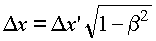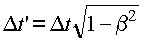2 Berkly's course of physics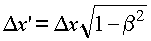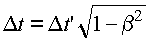3 L.D. Landau, E.M. Lifshits4 V.G. LevichIn this example, relativists sincerely do not understand the elementary difference: for the objectively existing world is not the same thing - "you are lengthened or shortened your twin" (to paraphrase a well-known anecdote about Napoleon and his long Marshal - not one and the same "rise Napoleon on his tiptoes, or shorten marshal a head "). And relativists, professing different views do not try to argue with each other (in fact, this is clan, or religion, but they are doing their best to play off opponents RT each other, often using more than minor differences).
So, for what of huge set of 2N variants are at war relativists as for next "the unique true doctrine"?

• 2. Limitation of Lorentz's transformations

• 3. Inconsistency of the relativistic concept of time
• We would remind that in classical physics results are obtained by one observer can be used by any other observer (i.e. it is true for all). Therefore, our goal is to formulate such a symmetric setting of a problem with results which are evident from the common sense. Relativists renounce the common sense permanently! Therefore, to prove the lack of contradictions and observability of relativistic effects, they must consider different results from the viewpoint of all different observers and compare all results between themselves. However, for some reason they never complete up the check to the end. The same cunning is observed at "formal reply" on necessity of use of diagrammes (though really someone will assert that Lorentz's transformations are insufficient, and diagrammes present something more?): relativists do not investigate the solutions about absence of contradictions from the viewpoint of all observers.
One more preliminary remark (though also obvious) concerns additivity and continuity of time (and and the inseparable connection of material objects with space and time). If the observer continuously moved on sites of a trajectory 1,2,3..., its age has changed as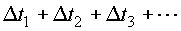Furthermore, the science of physics, as a rule, deals with objective measurable quantities. One manifestation of objectivity is that we can compare values of the same type, even if subjects over which measurements were not there at the same time (so that we can, in principle, to compare the weight of the German Shepherd with the weight of the brontosaurus, or the average life expectancy of driopithecus with the average life expectancy of the American 21st century, though they have never met each other). Therefore, when comparing travel on two different paths does not necessary not only to synchronize clocks (well, there is no such law in math that a comparison between numbers requires some pseudo-physical procedures invented by Einstein), but even so, these movements began or were simultaneously (on one trajectory someone could fly a billion years ago, and on the other trajectory - now, and the elapsed time can be compared.) Therefore, it is possible, for example, separately to compare the time spent in areas of accelerated motion of twins and separately to compare the time spent in areas of rectilinear uniform motion of twins.
We shall begin with the traditional paradox of twins. Many relativists involve acceleration of one of twins for its "explanation". We shall remind according to the SRT, that without acceleration, in opinion of each brother, another one should appear younger. However, we can see from Figure 1 that regions |OA| and |BC| with accelerations can be fixed equal in length. But for different cases i, we can change the distance |AB| of flight with a constant (on the module) big speed. For example, we can choose this distance in 3, 5 times more than the initial one, etc.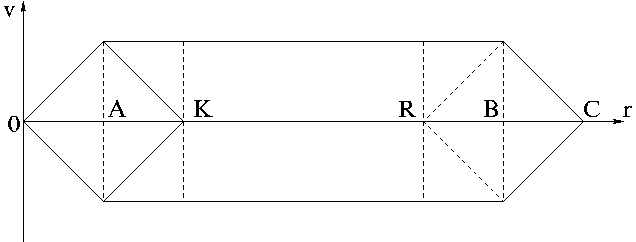It is clear that the same acceleration cannot explain a various difference in the age of twins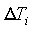for all these different cases. Moreover, the brother-homebody can be not too lazy and "take part" only in the accelerated movements at sections |OA| and |AK|, which are completely identical to the analogous sections for the brother-astronaut (starting at the calculated moment when the brother-astronaut will fly through the point R). Thus, the initial "explanation" of twins paradox by means of an acceleration, to which adhered Einstein, Pauli, Born and others, can be handed over in a "dusty archive" as not having any scientific value.
In the beginning we will prove the auxiliary statement (not having the direct relation to the SRT), that the presence of mutual accelerations of astronauts cannot lead to a difference in the time course. Most easier, it can be made by means of two identical trajectories-loops represented in Figure. Astronauts start from one point, and, being accelerated by identical means on the given loops up to identical large speeds, again fly through the initial point simultaneously. Naturally, owing to symmetry of a problem, changes in their ages during the acceleration will be identical, that the observer at the start point can confirm (this obvious sense of the result should be the same, regardless of a theory you believe in, and which you wish to be considered).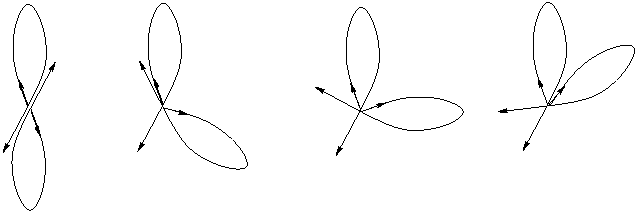We shall remind, that acceleration is a vector value. We can turn one of the trajectories-loops without change of its form at any angle around the start point. Though, in each such a case relative accelerations of astronauts will different, their ages in any of cases will be changed at the same value. This is a manifestation of the space isotropy. Obviously also, it is possible to transfer the given trajectories-loops in space in parallel (without form changes), and time "accretion" at such carrying over will not change. Similar identical trajectories-loops can be used for acceleration, for a turn and for deceleration of cosmonauts. Besides, accelerations can be chosen on value equal to acceleration of free falling on the Earth, that "the insignificant role of sites with acceleration" was clear even from the viewpoint of believing in the GRT.
Further, to these two loops we can attach identical rectilinear sections (vastly larger than the loops) for movement with an identical constant large speed. The identical U-turned loops can be attached to the ends of these sections. Thus, the equally accelerated astronauts (t1=t1') fly through one point after the termination of acceleration. After this, they fly with the same constant speeds. After the following equally turn (t3=t3'), they fly back with equal constant speeds and are slowed down by identical manner at the former trajectories-loops (t5=t5'). Owing to symmetry of the problem, the age of astronauts-twins will be obviously identical (common sense, which is called the "for life"; however, this result should be in terms of any other theory): TI=TII. But TI=t1+t2+t3+t4+t5, and TII=t1'+t2'+t3'+t4'+t5'. Again the trajectory of the twin-II can be turned at any angle relative to the twin-I around the start point O (see Fig.).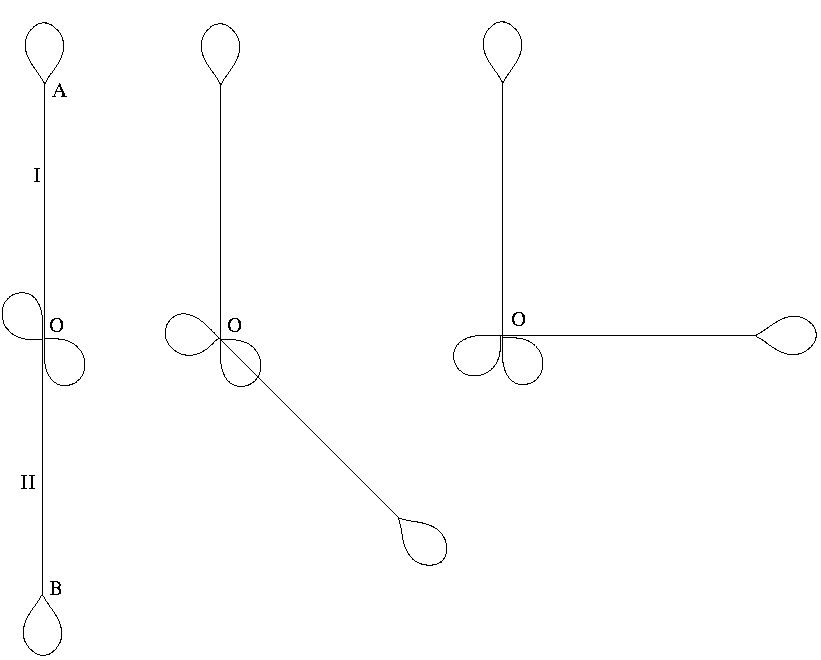Earlier we have shown that sites with acceleration (loops) do not influence on result of comparison of cosmonaut's age. Hence, it remains for sections of uniform motion to compare the values t2 with t2' and t4 with t4' only (and this problem "is purely the responsibility" of SRT). However, due to the vector character of velocity, their relative velocity will be changed at trajectory turn, that is according to the SRT from the viewpoint of each cosmonaut must be changed, that is obviously absurd (contradicts to the space isotropy). It is possible to prove discrepancy of relativistic concept of time even more shortly. Let for twins (two or more) point of start (the experiment beginning) - is the same, and let their meeting will take place in one point too. As only a square of relative speed is included into the formula of time dilatation, from the viewpoint of any of twins, it is already enough a nonzero (relative) speed of the other twin that the age of the other twin became less. And so should be from the viewpoint of any of twins that, obviously, it is impossible.
We will remind also the arguments of the president of the Royal astronomical society, professor Herbert Dingl: 1) According to the relativity principle itself, at separation and the subsequent reunion of the twins, it cannot have a place any observable effect showing presence of movement only one of them in the absolute image; 2) if after reunion one of two identical clocks participating in relative movement, will lag behind, it will testify to movement of only the given clocks; 3) thus, if the relativity principle is true, then indications of both clocks should be identical after reunion if they were identical before separation.
Numerous other logic contradictions of relativistic concept of time prove its full inconsistency also.

In the propagandas for the RT relativists make the following two deliberate forgeries. First, they deliberately try to create illusion as if time and clocks are the same thing. Long before the SRT-inventions, when the verified clocks from Europe have got on Equator, it has appeared that they began to lag behind strongly, and it were not mythical nanoseconds, but the values are practically appreciable for days. Thank God that to any idiot, if they then were, it has not come to mind that time for Equator goes "so essentially in another way". Scientists have defined the concrete physical mechanism influencing the concrete device of clocks at once. Accuracy of clocks, high or low, also has no relation to the course of time! Secondly, relativists deliberately try to create opinion, as if synchronisation of time and an exchange of signals in the course of experience is the same. So, at Gregorian calendar introduction, time synchronisation has been made. But anybody thus did not invent a time machine, did not fly to inform Alexander the Great, in what year B.C. he was born etc. Simply, if certain "the physical concept" applies for "scientific character", there are mathematical formulas and recalculation methods for it always. And synchronisation can be spent either before, or to time, or after experiment, or, in general, by a recalculation method. So, for all mental experiments of the SRT with two "objects" there exists biunique mathematical interconnection of classical representations with the relativistic ones. A.Poincare perfectly understood it, considering new concepts and transformations as one of (!) possible "agreements" only, on the same level (!) with former classical concepts (see in A.Poincare's book "About a science", work "Last thoughts", the chapter II and in Russian - comments in the end of the book in M.I.Panov, A.A.Tyapkin and A.S.Shibanov's article). But "new concepts" of the SRT are frequently give "misfires" at the description of spatial movement with number of objects more than two.

• 4. Inconsistency of the relativistic reduction of distances
• Since SRT was created as "a game with Einstein's light beams in absolutely empty space", any pseudo-paradoxes with use of an electromagnetic field (currents with contacts, lasers, light beams with mirrors etc.) can be easy resolved, and relativists slyly present them as allegedly lack of contradictions in SRT. For this purpose they simply make substitution and instead of real paradoxes "discuss" pseudo-paradoxes such invented or "added" by them with any electric contacts, allegedly effective explosions etc. So be attentive to such forgery! And now we proceed to some particular paradoxes of lengths shortening. Let a thin plate of large size lie on a solid plane. A small cross is cut out of the plate: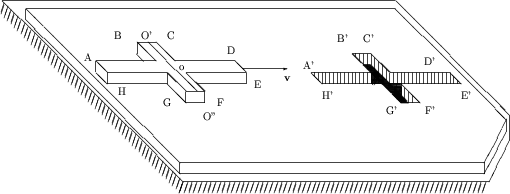Let the length of this cross be much larger than its cross-beam width |AD| >> |BC|. Let the cross slide horizontally over the plate, so that in classical physics it would just occupy its niche (fall into it under the effect of any force, for example, the force of gravity). We choose the relative velocity of motion v such that, in accord with relativistic formulas, the length to be shortened two-fold (or even more). Note that the center of gravity of the cross (point o) lies also at the cross-beam center. Hence, vertical motions of the cross (falling down, or turning over its front end) is possible only if: 1) center and the whole central line of a cross-beam (O'O") are over empty space, and 2) none of points C, D, E, F has support. From the viewpoint of an observer on the cross, he will slide over a two-fold shortened niche, since either the cross-beam and one of ends, or both ends of the cross lean against the plate. The known trick with turning of a rod (to which relativists persist in trying to reduce the length contraction paradoxes) fails in this case (this problem will be considered below). However, from the viewpoint of an observer on the plate, the cross (which became two-fold shorter) will fall down into the niche. Thus, we have two different events: does the downfall of the cross (a push against the plane) take place or not?
The following remark. Since the shortening of lengths of objects is associated with properties of space itself, the distance to objects must also be shortened (regardless of whether we approach the object or move away from it!). Therefore, if the velocity of a rocket is high enough (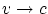), we can not only look at distant stars, but also "touch" them, because in our own reference system our own dimensions do not change. Besides, when flying away from the Earth for a long time (the value of acceleration is not limited by SRT), we will eventually be at the distance of just "one meter" from it. At which time instant will the observer at this distance in "one meter" see the reverse motion of the spacecraft (contrary to the action of rocket engines)?
Let's consider now the paradox of a sawn ruler (preliminary recollect a method of division of the whole to parts in the Galilee proof that there are no reasons for acceleration of free falling to increase twice at increase in mass of a falling body twice). Four identical rulers are represented in Figure. Ruler A lies motionlessly on start as the standard. Ruler B lies motionlessly on finish as the standard (both these rulers are represented only for presentation). Ruler C will move during the experiment. It is sawn on two equal parts 1 and 2. Ruler D will move during experiment too, but it is not sawn. We will consider movement of the first half-1 of ruler C separately: let it starts to move with uniform acceleration, reaches the big set speed V, flies with such constant speed and crosses the finish straight F by the right end. If the second half-2 of ruler C has started to move at the same moment as the first half-1, moved with the same uniform acceleration before achievement of the same speed V, and then flied further with the same constant speed, its site at the time of crossing of finish by the first half of a ruler - it will cross line O' as it is represented in Figure: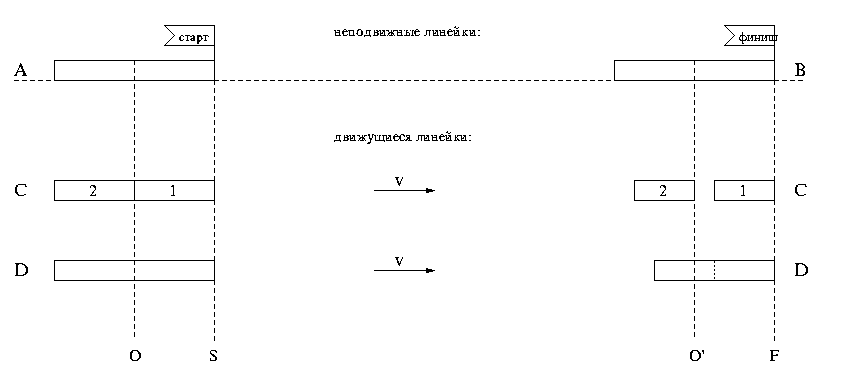This result is obvious, as the situation with the second half of a ruler differs from the situation with the first half of a ruler only by parallel translation of the beginning of coordinates (the right end of a half of a ruler from line S on line O). However, for not sawn ruler D the situation with finish will be quite other (the ruler is represented in Figure as reached finish as the whole). We have the logic contradiction. First, whence the ruler C could know, what it is sawn? Secondly, the saw cut of the zero value could not according to the SRT to turn to a nonzero spatial backlash. The contradiction cannot be eliminated even if one declares inequality of reference systems and necessity to have primary system with the defined arrangement of the beginning of coordinates (i.e. to offer the relativity!): after all the ruler can be sawn on 3 and more parts and rescuing one of saw cuts, the others saw cuts all the same form nonzero backlashes at movement.
Now we shall consider the spatial paradox connected with ostensibly existing relativistic turn of a rod. Let's remind the following problem. A thin rod of some length L flies along the axis X with a speed v, and the plate with a niche of the same size L runs with a speed V in a direction of the axis Z , so that in the classical case the rod will precisely pass through the niche (see Figure). Relativists "eliminate" contradictions in indications of different observers by introduction of relativistic turn of the rod. However, the situation with the relativistic angle of rod turning can elementarily be made dramatic one, since it uniquely depends on the ratio of speeds. Let the other smaller rod l slides with some speed on our rod. Observers on both rods will claim that the clearance between the rods is absent. However, according to the SRT and due to different speed of rods (v and v1), the big rod L and the small rod l should be turned at different angles relative to the plate for the observer on the plate. That is in the SRT the small rod will be turned upwards relatively the big rod, and there appears a clearance between the rods. We have the obvious contradiction.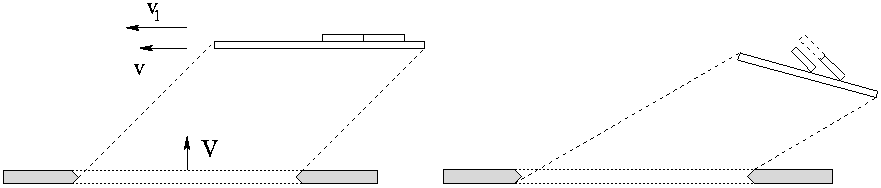This contradiction can be made even more vivid, if we will use the principle of division of a whole to pieces (firstly applied by Galileo to prove independence of acceleration due to gravity from weight of a falling body; see above the paradox of a sawn rule). Then if we will consider the rod l as a single whole, one situation (see Figure) turns out, as if the second half of the rod l is raised at some height above the rod L, on which there is a sliding. But if we will consider the small rod consisting of real two halves, then the given situation for the second half-rod is simply similar to the case of translation of origin and these halves appear with the forward ends on the big rod, but spatially divided (see Figure). Last situation is especially strange, since the cut of the zero size should remain zero at any turns or multiplications on the relativistic factor. Let's notice, that we have a little more "accompanied" to the SRT, having turned the small half-rods ABOVE the greater one. Actually, in the SRT there are no real firm bodies at all, impenetrable one for the other. All SRT formulas are derived for light flashes (model), but the latters are capable to pass through each other. As a result, to coordinate with indications of the observer at the rod center, it is necessary to assume, as if one rod passes through another one (absurd discrepancy of the model to reality).
Thus, representations of the SRT come to the contradiction with such an important and checked up element of relativity concept as the uniformity of space (a possibility of parallel translation of the coordinate origin). The presence of the other additional numerous logical contradictions makes to be impossible the constructive use of the relativistic concept of length.

• 5. Contradictoriness of the relativistic concept of "the relativity of simultaneity"
• Consider the mental experiment allegedly "demonstrating" the relativity of simultaneity: at the origins O and O' of reference systems S and S' that move relative to each other, a light flash occurs at the time of their coincidence. According to the SRT, during the time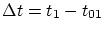on the clock of system S, the light will pass the distance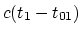from center O. For the same time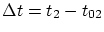on the clock of system S', the same light will pass the distance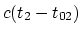from center O'. The time difference is not influenced by any adjustment of initial times, whether accomplished before the experiment, or after it by any method. It is possible to agree in advance about the flashes, recorded on the clock of system S (for example, periodically each million years), and "to organize" system S' for one instant before the flash occurs, selected in advance. Recall that the basic positive idea of the SRT consisted in the finiteness of the speed of interactions. The same idea is expressed by a short-range interaction theory, which reflects the field approach (via the Maxwell equations); namely: a light wavefront moving from a source to a receiver passes sequentially through all intermediate points of space. It is just this property that comes in a conflict with the notion of the relativity of simultaneity.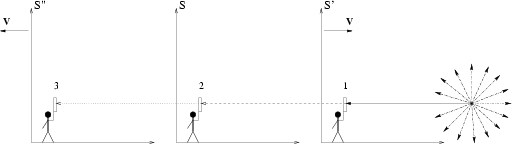To prove it, we use two statements from the SRT about observers moving each relative other: 1) one and the same light flash will reach two observers simultaneously despite the fact that the observers will spatially be separated by some distance during the light spreading; 2) kinematic formulas of the SRT (from textbooks) contain squares of velocities only. For example, let the first observer in system S' be moving towards the flash source at slow speed. Since the distance to the flash point is large (say a million light years), then for one million years both observers will separate from each other to a large distance. According to SRT formulas, the times of arrival of a signal will be the same for both observers. At what point of space did the first observer "pass" the light wavefront for the second observer? But what if he had held a mirror for the whole million years, and removed it one second before receiving a signal? In the second observer's opinion, the signal was reflected by the first observer somewhere ahead. But in this case what thing was reflected by the first observer, if none of his instruments did still respond to a flash? Similarly, a third observer can go away from the second one at the same velocity, but directed from the source. If the second observer held a mirror for a million of years except one second, would the third one see the light? On the one hand, since the SRT formulas include the square of velocity only, the second observer will consider the time of signal reception by the first and third observers to be the same. It can be agreed that when observers receive the signal under investigation, each of them will additionally send his own signal without delay. If second observer's calculations are correct, then since the problem is symmetric, he must receive the signals from the first and third observers simultaneously. On the other hand, according to Maxwell equations, the light propagates continuously, and the second observer will receive a signal from the first one simultaneously with the event, when he himself will see the signal under investigation. In second observer's opinion, at this time the light has still not reached the third observer. Thus, the second observer comes to a contradiction with himself: the first calculations by SRT formulas contradict the second calculations by the Maxwell equations. Obviously, the observers will see the flash sequentially, rather than simultaneously, since the spatial path of light is sequential: the source, the first observer, then the second and, at last, the third observer.
Thus, the relativistic "relativity of simultaneity" do not correspond to the Reality.

• 6. The relativistic equation of motion is reducing to the Newton one
• The relativistic equation of movement with the Lorentz force F can be elementarily written down as the classical second law of Newton with some other force F'. For this purpose it is necessary to find a derivative explicitly in the left-hand side of the relativistic equation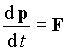and to multiply scalarly the left and right parts of the equation on v. Then, the expression follows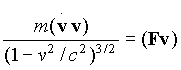.

Substituting it in the initial relativistic equation, it turns out the second law of Newton with the force F' at the right-hand side: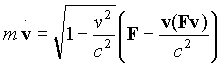.

Really relativists are not familiar with mechanics and hydrodynamics when in the most not relativistic area of speeds forces appear depending on speed of body movement (the concept of the attached mass etc. can be entered). Whether so there is something "great" in the similar "new" relativistic dependences of force on speed? Certainly - is not present! Formally, there can be any force in the given expression of force F' as a letter F. However, there are no proofs that the relativistic equation of movement can be applied to something, except to the charged particles being under an action of the Lorentz force. Thus, it is supposed that forces will be transformed at transition from one system to another one. Generally speaking, the idea that the same force can be different for different systems of observation is the flat nonsense for all experimental physics. Really, the way of writing arabic cipher on a dynamometer is independent on observer motion, i.e. readings of the dynamometer (fixing the force) will not be changed with observer motions. Any force acts between the "source" of this force and the concrete "object" of the applied force, and motion of some "strange eyes" has no relation at all (i.e. force can depends on the source properties, object properties, and their mutual motion). The experimental equations of motion with the initial conditions completely determine all characteristics of motion, including the firsts integrals of motion (the energy and momentum, which will be different from expressions for the model of non-interacting mass points).
Make now some remarks on the Maxwell equation. We consider in the beginning a "basic" question on invariance of the Maxwell equations, widely boosted in the SRT. In the Sivukhin's textbook [in Russian] the following four equations (in the differential form) are ascribed to the system of fundamental equations of electrodynamics: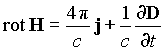,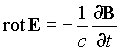,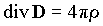,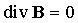.

However this system of eight equations (in the coordinate form) is obviously insufficient to determine 16 values (taking into account all components) E, D, B, H, j and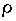. It is necessary also to introduce the medium characteristics into the equations. In view of existence of nonlinear, non-uniform, non-isotropic media, it cannot be made in the general case. It is possible to introduce some particular model representations about linear dependences in certain limits only: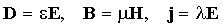and to add 9 more equations with three new unknown functions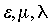(or constants - for model problems), describing the medium. There not exists uniform invariance of the last three equations in the general case. We shall remind, for example, about the existence of ferromagnets and ferroelectrics, for which the phenomena of a hysteresis are observed, that is the course of process depends on its pre-history. In such a case the behavior cannot be described by the differential equations at all. Whether it is possible "to inflate a soap bubble of the SRT" on invariance of a part from the full system of equations only? Obviously, no! So, it would be possible to extract any parts from any equation and to speculate on invariance of these items. Thus, the system of Maxwell equations in arbitrary media cannot be invariant under some unique physical transformation. First four equations can represent an independent interest in consideration of fields in emptiness only. However invariance of the Maxwell equations in emptiness under the Lorentz transformations means absolutely nothing for other phenomena. First, in empty space we can cut off half of piece and increase it twice - we shall obtain the same piece. Therefore, in empty mathematical space it is possible to use any systems of reference, consistent geometries and translating factors. It can be defined by convenience of the mathematical description only. However, the presence of real physical bodies and fields in space determines natural registration points, characteristic scales and interrelations between objects. All this defines differences of the real physical space from the empty mathematical space. Secondly, the property of some interactions to propagate in vacuum with the speed of light does not determine speed of propagation of these interactions in a medium. Despite of a huge role of electromagnetic interactions, disturbances in media propagate with the speed of sound. Knowing one constant c for vacuum, it is impossible to determine (for our "electromagnetic" world) speeds of sound and light in mediums: for example, light of not any frequency can propagate in substance (we shall remind about dispersion, absorption, attenuation, reflection). All these and many other properties (for example, anisotropy) fall outside the limits of applicability of the Maxwell equations in emptiness. Hence, to adjust all properties of the world under invariance of the Maxwell equations in emptiness is too overestimated claim of the SRT. Thirdly, the separation of the unique (in its action) field on electric and magnetic parts is conditional enough, and an invariance of these artificial items cannot have crucial importance. The important remark. The Maxwell equations themselves can gain some physical sense only, if the physical way of measurement of introduced field characteristics will be specified. At present, the equation of charged particle movement under action of the Lorentz force is reputed as such "a closing equation". Let's remind that during the different time periods the Lorentz force was not the unique form for an electromagnetic force. Among the well-known expressions there were: Ampere's force, Veber's force and other ones. If the modern electrodynamics had the self-consistent character (since fields are manifested on their power influence), then the expression for electromagnetic force should be deduced from the Maxwell equations, instead of to be artificially entered. Such an expression has been received by J.J.Smulsky, and it differs from the expression of the Lorentz force. Whether it is possible to consider the Lorentz force expression as the strict and consistent electromagnetic force on principle? Apparently, no! Though achievements of the modern electrodynamics are well-known, it is necessary to note some arguable moments too. First, even in the modern electrodynamics a braking by radiation is additionally introduced, but, however, it leads to senseless self-acceleration of charges (limited only postulatively by imposing conditions on values of fields). Secondly, the "origin" of quantum mechanics itself demonstrates that the Lorentz force does not describe adequately behavior of charges on scales of an atom. Thirdly, for the known phenomenon of the particle drift it is strange, that its speed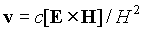appears independent on a charge, mass, and values of fields, but depending only on the ratio of fields E/H. Thus, the system of differential equations of the modern electrodynamics and its representations cannot be considered as strict and completely self-consistent on principle, capable to impose restrictions on other sections of physics.

• 7. The Michelson-Morley experiment
• SRT is known to rest upon two basic postulates: light-speed constancy, and the principle of relativity. So, we begin with the question, which is principal for the relativity theory: is light speed constant? Note that we cannot suppose in advance anything about the motion of the Earth: at Galileo's time, for example, such experiments would prove that the Earth was resting (generally speaking, before using a "device", the latter must be tested and graduated under laboratory conditions -- we must know what can be measured by it?). The answer to this question was seemingly given by the Michelson-Morley experiment. However, the term "constant" implies independence from time, spatial coordinates, light propagation direction, and, finally, characteristics of the light itself. An effort must be made to give an unprejudiced answer to the question: What matter could be identified in Michelson's interferometer? Notice that no speed is determined in the Michelson experiment at all, but some remainder of phases of rays is observed (and we can indirectly judge by the speed only). Recall that light was made to traverse two mutually perpendicular directions. We note also the following: To avoid the synchronization of timepieces at different points, both light beams traveled over a closed path; namely, in two mutually opposite directions. Therefore, only some "average" light speed, involving opposite directions, could actually be determined. Taking into consideration all the above mentioned, seemingly, Michelson's result can be stipulated as follows: mean light speed in two mutually opposite directions and at given frequency in some particular system is independent of the motion of this system. It is impossible to make the conclusion about any global constancy. At least two questions would remain in connection with the local result of the Michelson-Morley experiment all the same: depends or not the speed of light on spatial coordinates and time, and whether in vacuum the speed of light depends on characteristics of light itself?
The result of the experiment can be analysed both from the viewpoint of empty space (modern views), and with the viewpoint of presence of an aether (and different views on its properties). Light is known to behave in various phenomena as either a particle or a wave. At first, let us suppose light to possess a corpuscular nature. Then the Michelson - Morley interferometer may be represented as two mutually perpendicular arms with a single ideal reflector at the middle and two reflectors at the ends of the arms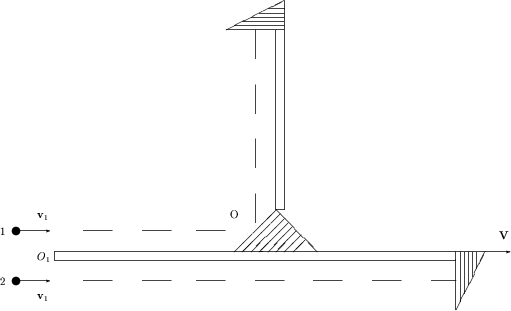Let the two particles moving parallel to each other at velocity v1 (relative to the "universal reference system") fall into the given set-up, which, in its turn moves at velocity V with speed v1 > V (relative to the same reference system). Then at point O1 the speed of particles relative to the set-up will be v1-V. After reflection at the set-up center, particle 1 will move in the perpendicular direction at the same speed v1-V relative to the set-up. The particles will reflect from the ends of arms simultaneously. Likewise, they will reach simultaneously both point O and point O1. No difference in speeds of these two particles for two mutually perpendicular directions will be observed, regardless of velocities v1 and V. Thus, if the light is supposed to be a flow of particles, then the experiments by Michelson - Morley (Kennedy - Thorndike, Tomachek, Bonch-Bruevich and Molchanov et al.) could not give any positive result.
Let us now suppose light possesses a wave nature. In this case, light speed can only depend on the properties of the propagation medium (ether or vacuum) and/or intrinsic characteristics of the propagating light itself (and, also as for a sound, it does not depend on speed of a source, but is added with speed of the receiver). If light is a wave, then only the light frequency changes with source velocity. So, for given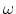, the light speed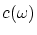does not depend on the source velocity. Here we have in mind the following situation: the light waves of the same frequency are identical to each other; and if we perceive the light of frequency, then it does not matter, whether it was emitted by a source at the same frequency, or if it was emitted at another frequency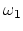, and due to source motion the frequency changed: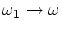(the Doppler effect). In both cases, the measured value ofis the same. Now we return to the Michelson - Morley experiment and similar ones by other researchers. Since the incident light, the light passed through the thin plate, and the light reflected from the mirrors, all have the same frequency in the same observation system, the light speedremained constant for the two mutually perpendicular directions, and the experiments could not detect anything. Thus, the attempt to find changes in light speed from the experiments with the same fixed frequency is wrong in itself. The only dependence we may try to discover is: all other dependences can enter only indirectly, through the Doppler effect.
If we accept a hypothesis that ether exists, then light speed depends on the properties of this medium (by analogy to sound). It is obvious then that light velocity cannot be added with source velocity (the boom from a supersonic aircraft propagates at the velocity fixed by the medium, and, as a result, the aircraft outstrips the sound). It is also obvious that, since light interacts with both matter (it scatters or absorbs) and ether (it propagates), then some interaction between ether and matter should also be observed. But in the Michelson - Morley experiment, something improbable was assumed; namely, a rigid "binding" of light to ether, along with absolutely no interaction of ether with bodies, (i.e. no ether entrainment by the Earth or by the interferometer). Imagine that somebody were create the following "theory": due to the Earth's axial rotation a constant wind with the value of about 400 m/s might be observed along terrestrial parallels. Measuring it by weathercocks with rotators, it would be obtained that the wind is permanently varying within the broad limits both in the direction and in the value depending on time and place. The "conclusion" would be made from this that the atmosphere is absent at the Earth at all. Of course, the theory would be complicated in the case of partial entrainment of the ether (and for some local experiments an ether entrainment can practically be complete inside the narrow boundary layer). However, this fact in no way disproves the ether hypothesis. One more remark, concerning the ether interpretation of experiments. For the sake of justice one should note that the Michelson experiment and its analogs (in spite of the disputes concerning the instrument structure and the theory) have always confidently, with allowance for possible errors, given a nonzero result. Only at instrument screening with a metal casing the result occurred to be close to zero one. We recall for the sake of objectivity, that all instruments are vacuumed now (i.e. made a locally closed system). And, for example, the local speed of sound in airplane's saloon will remain constant (independent on the wind outside) even at supersonic motion of an airplane. One of the well known ether viewpoint does not contradict the obtained results: Fresnel's entrainment for metal bodies is complete (Hertz's electrodynamics is valid for metals), and, hence, the ether is resting locally inside the metal casing relative to an instrument, and searching for the ether wind inside is senseless. Yet another moment is usually hidden by relativists. Even in the absence of metal casing, the presence of a thin glass plate (or air in the original experiments) leads to light reradiation from these local rest elements. As the result, in the ether concept the really measured velocity must be wittingly less than the velocity of orbital motion of the Earth. However there is the more serious objection to interpretation of the Michelson experiment, since from the ether point of view the analysis should be begun with the formulas used by Michelson: at their conclusion it was not considered the wave nature of light at all, in particular the Doppler effect and the aberration (that it would be possible to simulate for experiments with a sound in air). The conclusion appears the following: schemes with the fixed position of a source and the receiver concerning the fixed setup, moving as a whole in the environment, are not capable to find out change of the interferometric picture (inphase covering of waves) at change of orientation (turn) of the setup (the same situation Will be not only for light, but also in acoustics!).
Thus, the Michelson - Morley experiment does not witness the light speed constancy and does not testify against any classical principles.

• 8. The Ivese-Stilwell experiment and the Doppler effect for spherical waves
• Note that Ivese himself was a SRT opponent and explained the experiment from the ether theory viewpoint (it is characteristic of SRT to "put" everything into a personal "pile" in order to look more solid, pretending that if SRT "sinks", then "all science will also sink"). Generally speaking, unlike the elementary theory of the Doppler effect for plane waves, determination of a frequency dependence in some arbitrary configuration is a prerogative of experiments (and an implication of an additional hypothesis for time here is rather doubtful). Actually, the Ivese-Stilwell experiments, even in the ideal case (with neglecting real features of a process) would determine not the transversal Doppler effect, but the Doppler effect for two directions close to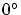and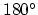, i.e. the effects close to longitudinal ones. These experiments are indirect, since the value of a relativistic correction is a calculated quantity (which is compared, in addition, from various regions, which results in the additional asymmetry). The additional experiments have shown essential systematic deviations from the relativistic expression (up to 60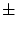10%). Therefore, the effect can be determined not so much by the Doppler expression, as by the feature of reactions in beams. We shall give some criticism of relativistic interpretation of considered experiments. Relativists describe the experiment in such a manner, as if the transversal Doppler effect is perceived from one point of a setup at some certain time instant (the time of passage through the middle perpendicular). Actually, the perceived signal is an integral sum from various regions of radiation for various time, and these regions are, in addition, not perpendicular to the motion (where, for example, the aberration has gone?). That is, the studied effect represents some "composite mean value" between two longitudinal Doppler effects. Besides, the theory (and the formulas) in SRT are presented for plane-parallel waves, but in fact we have point-like sources, i.e. the spherical waves at these distances. Consider a finite pulse signal. We write lengths of sides in a triangle: 1) the first side describes a way of the signal along the axis Y from the source to the origin of the reference system O, where the receiver was situated at the moment of emission of the signal: Y0=ct; 2) the second side describes a passed way of the receiver along the axis X from the moment of emission to the moment of the receipt of the signal: X1=vt'; 3) the third side (diagonal) describes a way of the signal from the source to the point of the receipt: ct'. Then, from the relation of sides in a triangle it can be found the change of a time delay as compared to the case at rest: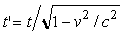. (In reality, change of time of signal receiving can be interpreted as the transversal Doppler effect for spherical waves which also exists both for light and in acoustics as well!) As a result, for the real source the displacement into the red area will be observed (a greater time of action of such a displaced line), and the effect should depend on the distance to the observation point. And who could prove that the classical Doppler effect for plane-parallel waves must be applicable for light? This effect possesses the classical form in the case of pure wave motion only, you know. But if light is not entirely a wave, other expressions could be obtained, including the relativistic ones. Thus, the given experiment can not be unconditionally attributed to the experiments confirming the relativistic time slowdown in SRT.
For the Doppler effect in the SRT relativists make the next forgery: they consider the dot light flashes (that is spherical waves!), but results compare to the classical effect of Doppler for plane-parallel waves. It is obvious that for plane waves no transverse Doppler effect exists (and it is not necessary here to relativists inflate cheeks). But if someone does not understand the difference between spherical and plane waves, then, apparently, he does not understand physics or mathematics (for example, at the level of the Soviet 8th grade middle school You can strictly find an exact solution of the following problem: an oscillating up and down float excites circular waves on the water surface; how many peaks of waves per unit of time will be fixed by an observer moving along a line over the water surface?).
In the beginning we will consider the spherical waves raised by a moving dot source in the environment (for example, it can be a sound or circles on water). Let a rest receiver of signals is placed at the point R. If a source was based at the point O all the time, the direction of signal's distribution would be represented by a line OR (the length of a wave can be defined, having divided distance |OR| into the number of accomplished oscillations in the time of passage of the given distance). The similar situation would be for a source which is based at some other point i. Let now the source move rectilinearly with a constant speed v. Let's choose conditionally a segment in length equals to the wavelength as an investigated signal and agree that we will watch a point corresponding to the beginning of this signal (for uniform movement it is quite equivalent it would be possible to watch the movement of the middle or of the end of this conditionally allocated segment). The source was at the point O at the moment of the beginning the signal sending, and the source is at the point i at the moment of the beginning the reception of the same signal by the receiver. Corner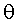is “a corner between velocity and the supervision line, measured in the receiver system” as it is usually in the theory of the Doppler effect.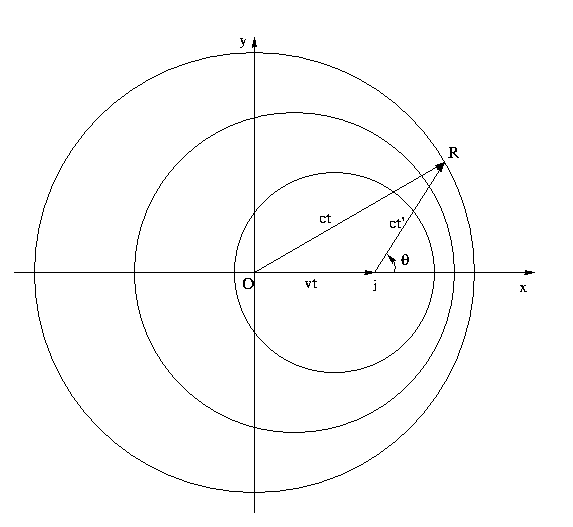It is easy to define the change of a period of perceived oscillations T' in comparison with the period T of oscillations for the source based at a point O - from interrelation of the sides of a triangle (distances, or, wavelengths - if the length of each side to divide into number N of the oscillations made during this time, where T=t\N, T'=t'\N). For this purpose we will take the theorem of cosines: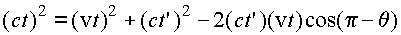. Solving this quadratic equation relative t', we have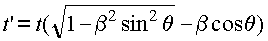, where as usually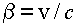. As a result, for the frequency shift it is received the following expression: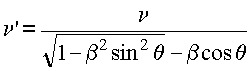.
Let now spherical waves are raised in the environment by a rest source i, and the receiver moves rectilinearly with a constant speed v, and the latter is in the point R at the moment of the beginning signal reception. The situation to this moment is represented in Figure: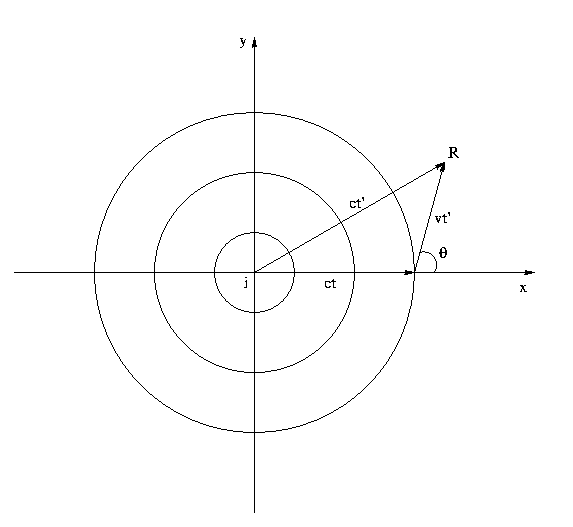Similarly, using the theorem of cosines for the given triangle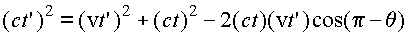, and resolving the quadratic equation relative t', we will find: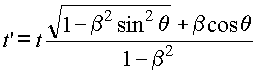. As a result the formula of the Doppler effect for spherical waves will look like:. Its correctness at any distances follows from the procedure of derivation of the formula. Actually a corneralso traces automatically distance between a source and the receiver, as, unlike a case of plane-parallel waves, this corner varies in the course of movement. First of all, in the received formula it is interesting for us the fact that there exists the transverse Doppler effect for spherical waves (if to substitute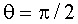in last formula of the Doppler effect), completely coinciding with the relativistic expression. At simultaneous movement of a source and the receiver the Doppler effect for spherical waves looks like: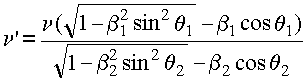. Now we will return to "ours relativists". Max Laue was the last among relativists, who wrote down one relativistic formula simultaneously including both movement of a source and a receiver. Then, possible, relativists have understood the contradiction of the simultaneous account of the both movements to the relativistic ideology itself, but not have agreed what of two formulas to leave (after all, A.Einstein used both ones!). As a result in the literature different authors have different formulas. Besides not clearly, how one relativistic formula of the Doppler effect could simultaneously give two classical formulas of the Doppler effect at limiting transition (after all, both of them are checked experimentally up and give the different observed results, for example, for sound)?

• 9. GRT - the "Great" theory of three and half observations
• Usually, even there exists a hundred different data, a theory is constructed not always: the data can simply be tabulated in a table. But in the case of the GRT we see "the Great theory of three and half observations", three of which are the fiction. Concerning the light deflection from rectilinear motion in a gravitational field, we should make the following statements. First, as it was pointed out by many experimentalists, a quantitative verification of an effect essentially depends on the faith of the concrete experimentalist. Second, even from the classical formula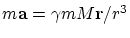it follows that any "object" (even of zero or negative mass) will "fall down" in the gravitational field. Third, with which a value does the effect be compared? With a value in empty space? As early as 1962, a group of Royal astronomers declares that the light deflection near the Sun cannot be considered as confirmation of GRT, because the Sun has an atmosphere stretching for a great distance. We would remind that the effect of refraction is long taken into account by astronomers for the terrestrial atmosphere. Lomonosov discovers the deflection of a light beam in the atmosphere of the Venus long ago. For explanation, imagine a glass sphere. Naturally, parallel rays (from distant stars) will be deflected to the center in it. Such a system is well known as an optical lens. The similar situation will take place for a gas sphere (the Sun's atmosphere). For accurate calculation of light beam deflection in the gravitational field, one should take into account the presence of the solar atmosphere and the fact, that density and temperature gradients on the beam path causes changes to the medium's refractive index and, hence, to the bending of the light beam. Even at the distance of a hundred of meters (near the Earth), these effects cause a mirage, so ignoring them for a beam coming from a star and passing near the Sun (for millions of kilometers) is a pure speculation.
The displacement of the perihelion of Mercury is, of course, a remarkable effect (but whether the sole example is sufficient "to attract" a scientific theory, or not?). The matter is that the Sun is not a solid body, and the motion of Mercury may cause a tidal wave on the Sun, which may in turn also cause a displacement of Mercury's perihelion. In any case, it is necessary to know the rate of transmission of gravitational interactions for calculating the effect of a tide due to the Mercury and other planets on Mercury's orbit characteristics, in order that the purely "gravitational" effect (if it exists) of the general relativity theory could be separated. Calculating the perihelion displacement in GRT (from the rigorous solution for a single attractive point), the impression is given that we know astronomical masses exactly. If we use GRT as a correction to Newton's theory, the situation is in fact opposite: there exists a problem knowing visible planet motions to reestablish the exact planet masses (to substitute the latters and to check GRT thereafter). Imagine the circular planet orbit. It is obvious in this case, that the Newtonian rotation period will already be taken with regard to an invisible precession, i.e. the period will be renormalized. Therefore, renormalized masses of planets (averaged by some way) are already included in Newton's gravitation theory. Since the GRT-corrections are much less than the perturbation planet actions and the influence of a non-sphericity, the reestablishment of exact masses can essentially change the description of a picture of the motion for this complex many-body problem. No such detailed analysis was carried out. Generally speaking, the situation with description of the displacement of the Mercury's perihelion is typical for relativist's behaviour. First, it was declared that the effect was predicted, but Einstein compared it with the well known results of approximate calculations, which was produced by Laplace long before origin of the GRT. Hope, each man understands a great difference between "predict" and "explain after the event" (remember the appropriate anecdote of Feynman). Second, there exists the most part of precession already in classical physics: the data of 19th century was found with taking into account influences of some planets. The result obtained was the value of 588'', whereas a deficiency in the calculated value make up about 43'' only, that is a small correction. (Note, that some data of 20th century indicate the total value of precession to be about 10 times higher than mentioned one, but the "deficiency for GRT" in 43'' is maintained - "taboo"; nevetheless, it could be a misprint and we will not cavil to 1/3 of "the great experimental base of GRT"). Third, the exact calculation for a many-body problem cannot yet be made even by the modern mathematics. In classical case the calculation was made as a sum of independent corrections from influences of separate planets (the Sun and planets were considered as material points). Naturally, the classical net result (more than 90% from observable one!) can some more be improved with taking into account the solar non-sphericity, influences of all planets (including small bodies) of the solar system, the fact that the Sun is not a solid object (a material point) and its local density in different layers must "follow" influences of other moving planets (this way of using real physical mechanisms can lead to obtaining the deficient small effect). But the relativist's declaration is inconceivable speculation! They "found" an effect (the small procent only!) considering motions of two material points only - the Sun and the Mercury. Sorry, and what will a correction be made with the GRT for the most part of the effect obtained classically? Do you fear to calculate? Then on what "a brilliant coincidence" do you repeat? It is the pure machination to a desired result!
We would remind two well-known experiments which were urgently ascribed by relativists to the GRT advantages. The Hafele-Keating's experiment consisted in the following: two pairs of cesium atomic clocks flew at an airplane in the east and west directions, and their readings were compared with the resting clock (in so doing the SRT "velocity effect" was taken into consideration, but its lack was proved above). The Pound-Rebka's experiment consisted in the following: using the Mossbauer effect, a frequency shift was detected for a photon which passed some distances in the vertical directions, both up and down. In physics it is not accepted to take into account the same effect twice. It is clear, that the acceleration and gravitation express some force, that influences various processes. But this will be the general result of the effect of namely the forces. For example, not any overload can be withstood by a man, the pendulum clock will not operate under zero gravity, but this does not mean, that the time stopped. Therefore, the rough Hafele-Keating's experiment states the trivial fact, that the gravitation and acceleration somehow influence the processes in a cesium atomic clock, and the high relative accuracy of this clock for a fixed site is fully groundless. Though the Hafele-Keating experiment was declared as confirming the GRT, this conclusion was made with use of a little sampling (again reduced). Other investigators, which had a free access to the primary data, made quite the reverse conclusion. Besides, the Hafele-Keating experiment was interpreted as the gravitation dependence of time (actually, the interpretation means change of the generator carrier frequency itself in the gravitational field). In such the case, however, it contradicts the interpretation of the Pound-Rebka experiment, where the generator was considered to provide the same frequency at any altitude (and some kind of these two experiments must be eliminated from the "GRT money-box"). The gravitational displacement is treated from the energy point of view as and in the classical physics, but where the relativity time slowdown in the gravity field has vanished in this case?
Many GRT inconsistencies are well-known: 1) the principle of correspondence is violated (the limiting transition to the case without gravitation cannot exist without introducing the artificial external conditions); 2) the conservation laws are absent; 3) the relativity of accelerations contradicts the experimental facts (rotating liquids under space conditions have the shape of ellipsoids, whereas non-rotating ones - the spherical shape); 4) the singular solutions exist. (Usually, any theory is considered to be inapplicable in similar cases, but the GRT for saving its "universal character" begins to construct fantastic pictures, such as black holes, Big Bang, etc.). To you to choose, whether it is necessary to be engaged in the similar "literary creativity"; at least, this "poetry" precisely does not concern a science.

• 10. Why all the same Newton?
(apologetics of classical physics)
• The classical concepts of physics were issued as a generalisation of thousand-year observations of diligent researchers over world around (including astronomical observations of the Universe). If the definition of relationships of the cause and effect for phenomena to be considered as one of the science purposes, then the important positive moment of the classical approach consists in separation of an object under research from the rest Universe. For example, a movement of eyes of the observer does not render appreciable influence on the concrete proceeding process in the overwhelming majority of cases (and especially on the all remained Universe). Certainly, there are "seeming effects", but one usually get rid of them by the graduation of devices, recalculation etc., to concentrate on the investigated process itself. The classical kinematic notions was actually introduced by Newton just for the determination of registration points and standards independent on the process under investigation. This founds the grounds for the common description of different phenomena, for the joining of various fields of knowledge and for the simplification of the description. Proceeding from practical demands of natural science, Newton understood that any creature is "excellently familiar" with the mentioned notions and practically uses" theirs (for example, insects that are incapable of abstract thinking in opinion of people). So, these notions are the basic ones, i.e. they cannot be defined through anything. Then, it is possible to give only an enumeration of "things" that will be meant by these notions or will be used in practice and to separate the abstraction that will be implied for idealized mathematical calculations. Because of this, Newton clearly separated absolute, true, mathematical time or duration (all these words simply are synonyms in this case!) from relative, seeming or ordinary time. Thus, time means the mathematical comparison between duration of the process under investigation and duration of the standard process. In classical physics the possibility of introducing the universal time has not been directly connected with the obvious restriction on the speed of signal transmission. More likely obtaining the universal time was connected with the possibility to recalculate it from local times with reasonable exactness. In perfect analogy to this, Newton separated the absolute space notion from the relative one, distinguished absolute and relative place, and distinguished between absolute and relative motions. A development of sciences shows that the classical kinematic concepts lead neither to internal logical contradictions nor to discrepancy with experiments. Also classical notions intuitively coincide with ones given to us in sensations: it is stupid not use they - it equals "to try to go by ears".
Whether has sense to discuss so-called kinematic effects (for example, a mythical curvature of space)? Certainly no! If to consider space to be crooking, it would be bent rulers in the same way and a similar curvature could not be revealed in any way. For this reason classical concepts are chosen ideal (and the most simple in practical use).
Whether impede classical concepts to "filling" of a real matter by any properties? Do not impede at all! Any property of a matter, which is discovered or can be discovered in the future, will be easily and organically included in the classical physics. For example, the discussion of possible properties of an aether is quite adequate in the classical physics (but it is no point to discuss an aether in the SRT, as it is deprived any observable properties in the SRT). Questions of generation of mass, charge etc. can quite be discussed in the classical physics. Whether the classical physics impede of potential possibility of existence for multilevel systems and movements (indefinitely "deep into" and "in breadth" and so various)? Too does not impede. Newton's first "law" speaks only about an ideal rectilinear movement of the allocated body which are not interacting with any other object. In the real Universe such a situation strictly is not presented (and only sometimes and approximately). Therefore, what type of natural movement (for example, movement on a circle or others) is realised in our unique Universe, it is entirely defined by existing real interactions.
Now about Newton's second law. An interaction of bodies leads to a change of the bodies' state. It is necessary to choose an "indicator" of this change. Newton chooses to observe body's acceleration as an "indicator" for change of its state of motion. Factually, the Newton second law presents a definition of the notion of "a force", and, from a standpoint of functional dependence, the force coincides with the acceleration within a dimensional factor (mass). Ideally, this way of a motion description (in the habitual form) must be written as ma=F(t,r,v). The relativistic equation of motion with the Lorentz force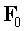can be written as the classical second Newton's law (see above) with the force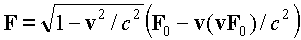. Though nature does not easily unravel its secrets for us, but it can be assured that the principle of the second law of Newton remains true: instead of the ideal expression for the force, we are obliged to use an expression that we found from experiments: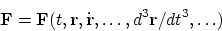. From this generalized expression it is seen that any derivative (including the second one) is not distinguished by anything, and only the experiment can determine the varieties of forces realized in the nature (recall, for example, the formula with an acceleration dependent force offered by Weber long before SRT). Whether the more "economical" record will be found for the movement description, other than Newton's second law, future will show (such an "improvement", generally speaking, is possible, but it not the question on the truth or incorrectness of the principle of the description itself!). The equations of movement completely (!) define the integrals of movement and they are not obliged to be the classical mechanical energy and impulse, obtained for noninteracting unstructured material points (we will recollect about classical examples with real dissipative processes, about hydrodynamic examples etc.).
In classical physics, there are not only relative values, but also absolute values (and it works well in practice), then it is already a more general theory than any theory that does not contain the absolute values.

• Still pair of remarks in small print.
• About larceny (plagiarism of ideas). This theme is discussed by many authors. Thieves' "logic" (apologetics) of relativists says: "charge A.Einstein in larceny of another's interesting ideas contradicts the statement about the R.T. inaccuracy." By no means is not present: if someone has stolen the car, and then it has broken, the fact of absence of the working car does not cancel the fact of its initial larceny. For example, ideas of the relativity theory contain in A.Poincare's earlier works, the SRT formulas are available in H.Lorentz's earlier works (And references to any one from A.Einstein's numerous predecessors has not given).
However distinction in interpretations of formulas is essential enough. For example, if Very Big the relativistic scientist will tell that he has compressed all Universe when went to the next bakery, a heap "Echoed" will confirm this bosh (to see these poor creatures did not read in the childhood a fairy tale "Naked king"). And after all for given so-called "the kinematic effect" is not present either the reasons, or the mechanism of its realisation (actions): from what all Universe was compressed if to go has climbed up in a head only to one person (even if he is "centre of the Universe")? Hence, the similar effect does not have a place in the physics (it only mathematical trick). At Lorentz, on the contrary, not each object was compressed concerning everyone at mutual movement (and especially not all space), but only the one who particularly moved through aether. Here there is also a reason (a start of motion with concrete speed through an aether) and the mechanism (interaction with an aether). Such effect already can quite be discussed in the physics. Difference of physics from mathematics consists in interpretations of formulas.

Once again we will remind that the given section gives short representation about directions of criticism of the relativity theory only, but does not substitute for itself more well-founded full versions of works. So it is necessary to read originals!